## Introduction

The theoretical work of Kane and Mele1 has marked a milestone in our understanding of the insulating phase of the matter. In their seminal work, they classified the bulk insulators according to the topological order of their electronic states using quantum metrics known as topological indices. When nontrivially ordered, it was predicted that such topological insulators (TIs) should exhibit gapless states at their boundaries, for example, at the surface or edges. The succeeding works have confirmed this prediction by theoretically proposing and/or experimentally discovering a number of two- and three-dimensional TIs, including HgTe quantum wells2,3 and uniaxially strained bulk HgTe4,5, a number of layered V2VI3 binary compounds, for example, Bi2Se3, Bi2Te3, and Sb2Te36,7, and the ternary compounds LaBiTe38, TlBiTe2 and TlBiSe29,10. The recent first-principles studies have further proposed that several half-Heusler compounds, such as LaPtBi, LuPtSb and YPdBi11,12,13,14, can turn into a TI under a uniaxial strain, similar to what was predicted and observed for HgTe4,5. Common to all these materials is the presence of heavy elements with a reasonably large atomic spin–orbit interaction (SOI). Such a similarity arises from the fact that in all TI systems, the bulk band gap is the result of band inversion by SOI4. In other words, without such a SOI-induced band inversion, no topological phase can be realized in a system.

In a more general context, SOI tends to lift the degeneracy of energy bands. In systems with inversion (I) symmetry, such a splitting leaves the energy states spin-degenerate, as long as the time-reversal (T) symmetry is hold. T-symmetry connects the states ψk,↑ and ψk,↓, whereas the I-symmetry enforces the degeneracy between ψk,↑(↓) and ψk,↑(↓). Breaking the I-symmetry lifts the latter constraint, and hence, lets the energy bands be spin-split at generic k-points. Rashba spin splitting (RSS)15 well exemplifies this situation, as described by HR=p2/2m[e·(s×p)], where e is the direction of the potential gradient, breaking I-symmetry, and s and p are the spin and momentum operators, respectively. This interaction leads to several unique phenomena, such as the spin Hall effect16, the spin Galvanic effect17 and the magneto-electric effect18. Furthermore, once superconductivity occurs in a Rashba system, unusual features such as the mixing of the singlet and triplet pair states, a large upper critical field beyond the Pauli limit19,20 and topological superconductivity with the Majorana edge channels21 can appear. As SOI is responsible for both RSS and non-trivial topology of electronic states in semiconductors, it is therefore of fundamental interest to know how they interplay with each other once they coexist in a system, and more importantly, what the impact of such an interplay is on the electronic structure of bulk and boundary states.

The purpose of this work is to study such an interplay in a layered polar compound, BiTeI. Backed by our earlier band structure calculations22,23, the angle-resolved photoemission spectroscopy measurements22,24 have revealed that the bulk conduction and valence states in BiTeI are subject to a giant RSS of the order of several hundred meV, lying among the highest reported so far. In this work we show, using the first-principles calculations, that by applying a reasonable hydrostatic pressure, P, the material turns into a TI with many interesting features, such as a nearly double enhancement in RSS accompanied with an unconventional metallic behaviour at the quantum phase transition. It is also demonstrated that unlike the I-symmetric TIs, the gapless Dirac states in the TI phase of BiTeI have completely different shapes at different sides of the material. Consequently, here the surface states on both sides can interestingly have the same spin helicity in a rather broad range of energies inside the bulk band gap, in sharp contrast with the centrosymmetric TIs.

## Results

### Basic properties

BiTeI belongs to the trigonal space group of P3m1. As shown in Fig. 1a and b, the crystal structure of BiTeI has a non-centrosymmetric layered structure along its crystallographic c axis with three atoms in one unit cell. Within each unit, a Bi atom is sandwiched between one Te and one I, forming a triple layer. Due to the strong covalency and ionicity of Bi–Te and Bi–I bonds, respectively, the bulk crystal intrinsically possesses a polar axis along the z direction. Despite the strong chemical bonding within each triple layer, the adjacent triple layers are weakly coupled via the Van der Waals interaction. From our previous calculations23, we know that around the Fermi level EF, all the bands are essentially p-type, with conduction bands dominated by Bi-6p and valence bands composed of Te-5p and I-5p states. Moreover, due to the negative crystal-field splitting (CFS) of the valence bands and positive CFS of conduction bands near EF, in the absence of SOI, the top valence bands (TVBs) and the bottom conduction bands (BCBs) both become pz type (to avoid any confusion they are referred to as pzA and pzB, respectively). These features make the electronic structure of BiTeI similar to that of the well-known TI systems Bi2Te3 and Bi2Se3 (ref. 6). However, here, because of the absence of I-symmetry, it is not possible to assign a distinct even or odd parity to each band. It is also worth noting that in the case of BiTeI, the minimum energy gap EG is not at the Brillouin zone (BZ) centre Γ, but near the hexagonal face centre of the BZ, A point, where kx=ky=0 and kz=π/c, as shown in Fig. 1c. As will be shown below, because of the latter difference, BiTeI in TI phase shows a rather different topological order from what has been found for Bi2Te3 and Bi2Se3. Introducing SOI, both spin and orbital mixings are allowed. Consequently, pzA (pzB) transforms to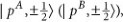thereby getting energetically repelled upward (downward). This accordingly closes the band gap from 1.2 eV down to 0.286 eV and induces a giant bulk RSS among these two sets of bands around point A (ref. 23). Despite such a huge reduction in EG, BiTeI remains a trivial insulator as EG still originates from the atomic orbital hybridization between Bi and its neighbouring Te and I atoms. The respective band diagram is shown in Fig. 1d.Figure 1: Crystal structure, BZ and schematic diagram of band splitting.

### Topological phase transition in BiTeI under pressure

Our strategy to turn BiTeI into a TI is to modify its chemical bonds by applying an external hydrostatic pressure. As schematically shown in Figure 1d–f, through this modification, we can effectively control both CFS and SOI such that at a critical pressure Pc,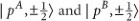become degenerate, whereas at higher P's their energy ordering is reversed, thereby forming an inverted band gap. Controlling CFS by P is rather easy to understand, because any change in the Bi–Te and Bi–I bonds leads to a change in the energy splitting of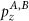andstates. For example, our non-relativistic band structure calculations reveal that at Pc, the CFS of TVBs is so enhanced that, EG is reduced by 200 meV (see the Supplementary Fig. S1). Any band-gap narrowing associated with the CFS modification can be further enhanced through a subsequent enhancement of RSS ofandstates. As described in detail in Bahramy et al.23, this is due to the fact that these two states are symmetrically of the same character, and hence, can very effectively couple with each other through a Rashba-type Hamiltonian if they are energetically close to each other. In other words, the closer they are to each other, the larger RSS would be achieved.

To elucidate this mechanism, we show in Fig. 2a–c the respective electronic band dispersions of TVBs and BCBs along the high-symmetry direction HAL of BiTeI as hydrostatically compressed by V/V0=1, V/V0=0.89 and V/V0=0.86, where V0 corresponds to the lattice volume at ambient pressure, Pambient. As shown, at Pambient (V/V0=1), a comparable giant RSS can be seen for both sets of bands with an EG of 286 meV. The corresponding Rashba energy ER, defined as the energy difference between the conduction band minimum (CBM) and the conduction band crossing point, is nearly 110 meV, in perfect agreement with that observed by the angle-resolved photoemission spectroscopy measurements22. Compressing the volume down to V/V0=0.89, ER monotonically increases until it reaches 200 meV, astonishingly about two times larger than that at Pambient, as shown in Fig. 2b (for a detailed comparison, see Supplementary Fig. S2 also). At this point, the system reaches its quantum critical point, represented by a full band gap closing along the AH directions. It is worth noting that such a quantum phase transition in BiTeI differs from that in usual centrosymmetic TIs, as there, it is mediated through a band gap closing at a single high-symmetry k-point, for example, the Γ point25,26, whereas in BiTeI, as will be shown shortly, due to the spin splitting, the band gap is closed at six k-points, all along AH directions. Thus, they are describable by six 2×2 Dirac Hamiltonians. Here it is important to stress that because of the T-symmetry, these six k-points are two-by-two paired with each other; therefore, their corresponding states form three pairs of 2×2 Dirac fermions. As the band touching is mediated through odd number of such pairs, the transition to TI phase is in principle allowed. Further increasing P, ER starts decreasing and an inverted band gap emerges between the TVBs and BCBs. Figure 2d–f demonstrates the orbital characteristics of BCBs and TVBs at V/V0=1, V/V0=0.89 and V/V0=0.86, respectively. A thorough analysis of these bands reveals a clear change in their atomic orbital characteristics as a function of V/V0. Although at V/V0=1, the BCBs and TVBs are dominated by Bi-6p and Te-5p, respectively, at V/V0=0.89, they appear to share rather the same orbital characters near the band touching point. On the other hand, at V/V0=0.86, one can clearly see that Bi-6p orbitals now contribute more significantly to TVBs, whereas the BCBs become strongly dominated by Te-5p and I-5p states, a clear indication that a band inversion has happened, and hence, the system is now in the TI phase. It is worth adding that in other parts of BZ, including the plane encompassing Γ, M and K, the band gap remains finite for the whole range of P's.

To make sure that BiTeI for P>Pc becomes a TI, we have calculated the so-called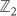topological invariant of the whole system when hydrostatically compressed by V/V0=0.86. By definition, for three-dimensional systems, thetopological invariant is determined by four indices, among which one is called the strong topological index ν0, and the three others are weak topological indices ν1, ν2 and ν34. The complete list is then described as=ν0; (ν1ν2ν3). For a normal insulator, all the four indices are zero, whereas a strong TI is characterized by ν0=1. If ν0=0, but any of ν1–3 is 1, the corresponding system is called a weak TI. For the numerical computation of theinvariants, several approaches have been proposed27,28,29,30. Here we follow the approach introduced in Soluyanov and Vanderbilt27 (see the Supplementary Methods and Supplementary Fig. S3 for a full description of our computational method for the calculation of thetopological invariants). Our calculations show that at V/V0=0.86, BiTeI belongs to=1; (001). This clearly indicates that it is a strong TI. Interestingly, the corresponding ν3 turns out to be 1, which can be attributed to the band touching in the hexagonal face of BZ with kz=π/c, encompassing A and H points. The interference between the physics of strong and weak TIs could be therefore an interesting problem here. For example, ν3=1 means that the one-dimensional conduction channel can appear by dislocations with a Burger's vector along the z axis31. Thus, the present system may offer an ideal medium to study the interaction between the surface Dirac fermion as guaranteed by ν0=1 and such a 1D channel.

To further confirm that BiTeI becomes a TI for P>Pc, we next show in Figure 3 the surface band structures corresponding to the I- and Te-terminated sides of BiTeI. Although the surface states are fully gaped at Pambient, a gapless state appears for both sides at Pc. At higher pressures, as shown for the case of V/V0=0.86, gapless surface states appear within the bulk band gap. A clear indication that BiTeI has now become a strong TI, thereby further confirming the results of ourinvariants calculations. Interestingly, the shape of the Dirac surface states at the Te-ended side of BiTeI completely differs from that at the I-ended one. Although for the former, the Dirac point is deeply buried inside the energy valley formed by the Rashba-split TVBs (resembling the Dirac surface states in Bi2Te3), on the other side, the Dirac point is energetically well above the bulk CBM. By increasing P, due to the reduction of RSS and the subsequent increase in EG, the latter Dirac point becomes energetically closer to CBM. We expect this to be a characteristic feature for all non-centrosymmetric TI candidates, making them distinguishable from I-symmetric TI systems. As a consequence, in a non-centrosymmetric TI, the electrons are fractionalized into nonequivalent halves on the top and bottom surfaces. This would lead to some novel features and new spintronics functionalities. As shown in Figure 3g and h, the surface Dirac fermions show the similar spin polarization patterns at the top and bottom surfaces, in sharp contrast to the centrosymmetric TIs. It is to be noted that for some thin slabs of Bi2Se3 and Bi2Te3, such an effect appears to happen when the equivalence of the two surfaces is lifted, for example, by applying an electric field32 or by differing chemical functionalization33. Because of this effect, the Dirac point traverses across the bulk band gap on going from the top side to the bottom side, and always crosses EF on the side surface as long as EF is within the bulk gap. Therefore, if one applies a magnetic field or dopes magnetic impurities, an insulating stripe is expected to appear on the side surface (as EF is now located inside the energy gap of the side surface Dirac fermion). Also, the giant spin Galvanic effect is expected, as the current–spin relation of the top side is same as that of the bottom side. When the hybridization between the Dirac fermions at the top and bottom surfaces occurs, in the momentum space, a gap appears along a one-dimensional (nearly) circular path, resulting in the divergence of the density of states. This accordingly leads to the enhanced electron correlation effect, and consequently, to the excitonic instability. These are just a few examples of the novel phenomena expected in the non-centrosymmetric TIs.

### Estimation of Pc

Let us now briefly discuss on the possible value of Pc required for topological phase transition. Performing two sets of volume optimization calculations using the local density approximation (LDA) and generalized gradient approximation (GGA), and then fitting the respective free energies E(V) to the Murnaghan equation of state34(see Fig. 4), we estimate the upper and lower limits of the bulk modulus B0 of BiTeI to be ~21.9 and ~8.9 Gpa, respectively. The respective LDA and GGA values of the pressure derivative of the bulk modulus B0 are similarly found to be 7.7 and 7.8, respectively. As mentioned above, our calculations indicate that at Pc, V is compressed by 11%. Using the relation34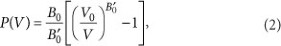Pc is accordingly expected to be in the range of 1.7–4.1 GPa. Given that this range of P's is rather easily affordable in the laboratory, we thus hope this work would stimulate experimentalists in this field to explore such an intriguing topological insulating phase in BiTeI.

## Discussion

At this point, it is worth explaining why BiTeI becomes gapless only at a certain Pc, but not for a range of P's as discussed before35,36 and why such a gapless state occurs along a specific direction. Group theory is the key to answer these questions. Let us first explain the role of crystalline symmetry for the development of dispersion minima (maxima) at BCB (TVB) along particular directions. As already mentioned, BiTeI belongs to C3v symmetry consisting of a three-fold rotation C3 along the z direction and three mirror operations M: y→−y, where y is along the AH directions. For spin 1/2 electrons, C3 and M can be represented as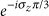and iσy, respectively, where σx,y,z are the Pauli matrices for spin degrees of freedom. Additionally, T operator can be defined as iσyK, where K is the complex conjugation. We can then construct a two band Hamiltonian Hc(k) for the BCBs invariant under C3, M and T. Up to cubic terms of k, it turns out to be:where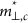and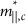are the in-plane and out-of-plane effective masses of the BCBs and νk,c=νc(1+αck2), respectively. Note that in a similar manner, one can construct Hv(k) for the TVBs. The third term in Hc(k) is obviously the Rashba term allowing a cylindrical in-plane spin splitting within the kx,y plane. The fourth term, hereafter referred to as Hw(k), acts as a warping term, trigonally distorting the energy bands.

Because of Hw(k), the inner and outer branches of the Rashba-split conduction bands are distorted in a way that by approaching CBM, they first merge together at the k-points along the AL directions and then form six energy pockets, each of which is centred along one of the six AH directions, as shown in Fig. 5a. Exactly at CBM, these six pockets reduce to six points. In the same manner, Hw(k) distorts TVBs such that the valence band maximum appears as a point similarly lying along AH. This accordingly explains why band touching between TVBs and BCBs occurs along the AH direction at Pc. It is to be noted that as a direct result of Hw(k), the energy gap along the AL directions is always larger than that along the AH directions for the whole range of P's, as shown in Fig. 2 and also in the Supplementary Fig. S2(e). Accordingly, neither at Pc nor at any other P the band touching can happen along the AL directions. The effect of P is to enhance λ for PPc, such that at the band-touching points, one can clearly see a rather large gap (as large as 40 meV) along the AL directions where the Hw contribution is zero. Such a warping effect and its enhancement at the quantum critical point can be well understood by comparing Figure 5b–d, in which the isocontours of energy for an arbitrary EF fixed at 20 meV above the CBM (corresponding to the dashed brown lines in Fig. 2) are shown at different P's. As can be seen, at Pc, the inner and outer branches of the Rashba-split conduction bands are just about to form the six energy pockets, whereas away from Pc, they form two distinct rings among which the outer one appears to be more significantly distorted by Hw.

Let us now address the first question, that is, why the gapless state in BiTeI can be realized only at a certain Pc, but not for a range of Ps. For the description of gap-closing, we can focus on the two bands touching at Pc among the four bands near the chemical potential. Generally, the topological phase transition in time-reversal invariant, non-centrosymmetric systems can be described by using a two-band Hamiltonian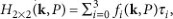where τ1,2,3 are the Pauli matrices and τ0 is the unit matrix. The real functions f0,1,2,3 depend on the three momenta k=(kx, ky, kz) and P, and are assumed to take into account the interaction of conduction and valence bands. The topological phase transition through an accidental band touching occurs if and only if the three conditions of f1,2,3(k, P)=0 are satisfied simultaneously in the (k, P) space. According to the recent work by Murakami,35 and Murakami and Kuga36, the band touching points, in general, form a curve in the (k, P) space, because the three conditions f1,2,3(k, P)=0 cannot uniquely specify the four parameters (k, P). Therefore, if the system is free of additional constraints other than the time-reversal symmetry, a gapless metallic phase is expected to appear in a finite range of PЄ(Pc1, Pc2) between the two critical pressures Pc1 and Pc2. The occurrence of the single Pc in BiTeI is traced back to the fact that the band touching occurs along a particular direction in BZ on which the Hamiltonian has an additional symmetry constraint. Along the AH line, for example, (kx, kz)=(0, π/c), the system is invariant under the combined operation Ω≡TM of the T and M symmetries. For T=iσyK and M=iσy, Ω is given by K, which imposes the following reality conditions:Because of this reality condition, f2(ky, P)=0 at all points along the AH direction. Therefore, the band touching can be achieved when the two conditions f1,3(ky, P)=0 are satisfied in the (ky, P) space. As the number of conditions to be satisfied is the same as the number of parameters, a gapless phase appears only at a single critical point (ky,c, Pc).

To conclude, using first-principles calculations we have examined the role of spin–orbit interaction in the giant bulk Rashba semiconductor BiTeI and found that under the application of pressure, it leads the system to be an unusual topological insulator. The novel surface states and quantum critical phenomena are intriguing problems for further studies. The effects of the long-range Coulomb interaction and disorder are, for example, the two important issues remaining to be addressed by both the theory and experiment.

## Methods

### Volume optimizations

To simulate the effect of P, we optimized the structure of BiTeI crystal at various volumes ranging from V/V0=1 to V/V0=0.84, where V0 denotes the volume of BiTeI unit cell at Pambient (a=4.339 ˚ and c=6.854 ˚). For a given volume, both the atomic positions and crystal's shape were allowed to be fully optimized until the magnitude of force on all ions became less than 0.005 eV ˚−1. All the structural optimization calculations were performed using both the LDA functional and GGA functional of Perdew-Burke-Ernzerhof (GGA-PBE) as implemented in the VASP programme37,38. The corresponding BZ was sampled by a 20×20×20 k-mesh. The respective upper and lower limits of bulk modulus B0 was estimated by fitting the LDA and GGA–PBE-free energies E(V) to the Murnaghan equation of state34, using equation (1). To relate the volume changes to P, we then used equation (2).

### Bulk electronic structure calculations

Within the same level of the GGA–PBE theory, the electronic structures of the optimized structures were calculated using the augmented plane wave plus atomic orbitals method as implemented in the WIEN2K programme39. For this calculations, the muffin tin radii were set to RMT=2.5 bohr for all the atoms and the maximum modulus of the reciprocal vectors Kmax was chosen such that RMTKmax=7.0.

### Surface electronic structure calculations

To calculate the surface band structure, for each V/V0, the corresponding augmented plane wave plus atomic orbitals Hamiltonian was first downfolded into an effective low energy 18×18 tight-binding model, using maximally localized Wannier functions40,41,42. We chose px, py and pz states as the projection centres on all I, Te and Bi atoms to span the top 12 valence bands and the 6 lowest conduction bands by our 18×18 models. On the basis of these realistic models, we then constructed large tight-binding supercell Hamiltonians composed of 50 units of BiTeI along its hexagonal c axis. The surface states of each side is then computed by diagonalizing the whole Hamiltonian and projecting the corresponding eigenstates onto the maximally localized Wannier functions of the corresponding surface layer.